Courses

# Extra Questions and Practice Exercise- Rational Numbers Class 8 Notes | EduRev

## Class 8 : Extra Questions and Practice Exercise- Rational Numbers Class 8 Notes | EduRev

The document Extra Questions and Practice Exercise- Rational Numbers Class 8 Notes | EduRev is a part of the Class 8 Course Class 8 Mathematics by VP Classes.
All you need of Class 8 at this link: Class 8

Question 1: Multiply the reciprocal of (7/8) by the reciprocal of (-2/21)
Solution: Given two rational numbers 7/8 and -2/21

We know,
Reciprocal of (-2/21) is (-21/2)
Reciprocal of (7/8) is (8/7)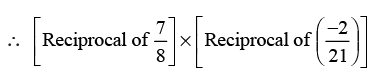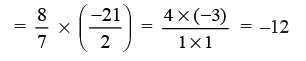Question 2: The product of two rational numbers is (-28/75). If one of the numbers is (14/25), find the other.
Solution:
Product of two numbers = -28/75
Any one of the rational numbers = 14/25
Let the other number be R
∴ R х 14/25 = -28/75
The other number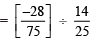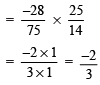Thus, the required rational number is (-2/3)

Question 3: Find three rational numbers between –3 and –4.
Solution: By formula method,

a = -3 and b = -4
Therefore, the rational number b/w -3 and -4 is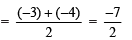A rational number between (–3) and (-7/2)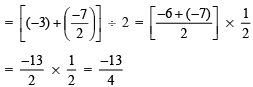A rational number between (-7/2) and (-4)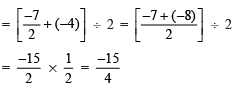Thus, the three rational numbers between (–3) and (–4) are -7/2, -13/4, -15/4.

Question 4: Find three rational numbers between (1/2) and (–2)
Solution: By formula method,

a = 1/2 and b = -2
A rational number between  (1/2) and (–2)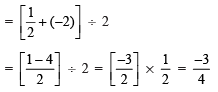A rational number between 1/2 and (-3/4)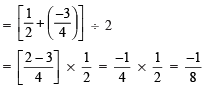A rational number between (-3/4) and (–2)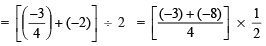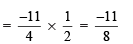Thus, the three rational numbers between 1/2 and -2 are -3/4, -1/8, -11/8.

Offer running on EduRev: Apply code STAYHOME200 to get INR 200 off on our premium plan EduRev Infinity!

93 docs|16 tests

,

,

,

,

,

,

,

,

,

,

,

,

,

,

,

,

,

,

,

,

,

;